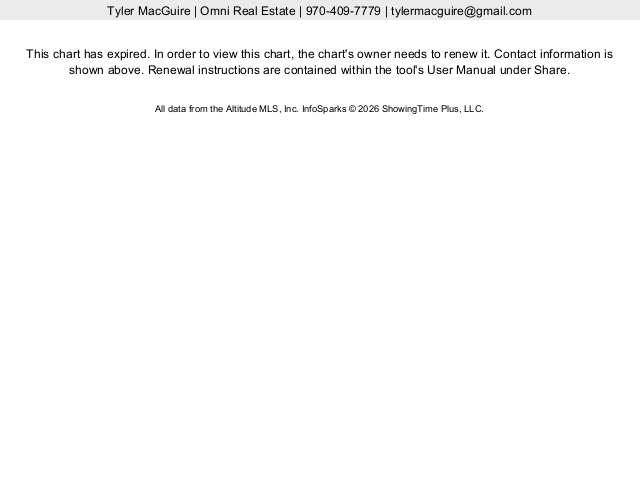Your Guide to Summit County Real Estate
• Price

• Type

## Income & Commercial

• Beds

• Baths

• More
•  Days on Site: All 1 Day 2 Days 3 Days 4 Days 5 Days 6 Days 1 Week 2 Weeks 1 Month Price Change: All 1 Day 2 Days 3 Days 4 Days 5 Days 6 Days 1 Week 2 Weeks 1 Month Sale Type: All Foreclosures HUD Homes Short Sales No Short Sales Square Feet: No Preference 10,000+ 9,000+ 8,000+ 7,000+ 6,000+ 5,000+ 4,000+ 3,500+ 3,000+ 2,750+ 2,500+ 2,250+ 2,000+ 1,800+ 1,600+ 1,400+ 1,200+ 1,000+ 800+ 600+ 400+ 200+ Year Built: No Preference 2020+ 2019+ 2018+ 2017+ 2016+ 2015+ 2014+ 2013+ 2012+ 2011+ 2010+ 2009+ 2008+ 2007+ 2006+ 2005+ 2004+ 2003+ 2002+ 2001+ 2000+ 1999+ 1998+ 1997+ 1996+ 1995+ 1994+ 1993+ 1992+ 1991+ 1990+ 1989+ 1988+ 1987+ 1986+ 1985+ 1984+ 1983+ 1982+ 1981+ 1980+ 1979+ 1978+ 1977+ 1976+ 1975+ 1974+ 1973+ 1972+ 1971+ 1970+ 1969+ 1968+ 1967+ 1966+ 1965+ 1964+ 1963+ 1962+ 1961+ 1960+ 1959+ 1958+ 1957+ 1956+ 1955+ 1954+ 1953+ 1952+ 1951+ 1950+ 1949+ 1948+ 1947+ 1946+ 1945+ 1944+ 1943+ 1942+ 1941+ 1940+ 1939+ 1938+ 1937+ 1936+ 1935+ 1934+ 1933+ 1932+ 1931+ 1930+ 1929+ 1928+ 1927+ 1926+ 1925+ 1924+ 1923+ 1922+ 1921+ 1920+ 1919+ 1918+ 1917+ 1916+ 1915+ 1914+ 1913+ 1912+ 1911+ 1910+ 1909+ 1908+ 1907+ 1906+ 1905+ 1904+ 1903+ 1902+ 1901+ 1900+ 1899+ 1898+ 1897+ 1896+ 1895+ 1894+ 1893+ 1892+ 1891+ 1890+ 1889+ 1888+ 1887+ 1886+ 1885+ 1884+ 1883+ 1882+ 1881+ 1880+ 1879+ 1878+ 1877+ 1876+ 1875+ 1874+ 1873+ 1872+ 1871+ 1870+ 1869+ 1868+ 1867+ 1866+ 1865+ 1864+ 1863+ 1862+ 1861+ 1860+ 1859+ 1858+ 1857+ 1856+ 1855+ 1854+ 1853+ 1852+ 1851+ 1850+ 1849+ 1848+ 1847+ 1846+ 1845+ 1844+ 1843+ 1842+ 1841+ 1840+ 1839+ 1838+ 1837+ 1836+ 1835+ 1834+ 1833+ 1832+ 1831+ 1830+ 1829+ 1828+ 1827+ 1826+ 1825+ 1824+ 1823+ 1822+ 1821+ 1820+ 1819+ 1818+ 1817+ 1816+ 1815+ 1814+ 1813+ 1812+ 1811+ 1810+ 1809+ 1808+ 1807+ 1806+ 1805+ 1804+ 1803+ 1802+ 1801+ 1800+ Garage: No Preference 1+ 2+ 3+ 4+ 5+ 6+ 7+ 8+ 9+ 10+ Levels: No Preference 1 Level 2 Levels 3 Levels 4 Levels Lot Size: No Preference .15+ acres .25+ acres .35+ acres .5+ acres .65+ acres .80+ acres 1+ acres 1.25+ acres 1.5+ acres 2+ acres 2.5+ acres 3+ acres 3.5+ acres 4+ acres 5+ acres 10+ acres 20+ acres 30+ acres 40+ acres 50+ acres 100+ acres 150+ acres 200+ acres 250+ acres 300+ acres 350+ acres 400+ acres 450+ acres 500+ acres Style: No Preference Cabin/Cottage Contemporary/Modern Mobile Home Multi-Level Ranch Victorian Sort: No Preference Price - Low to High Price - High to Low Year Built - Low to High Year Built - High to Low Sq. Feet Lot Size \$ Sq. Foot New Listings First

Save Search
More Less
Map List Gallery
Aspens at Eagles Nest Homes For Sale

Here you will find all Aspens at Eagles Nest homes for sale as well as statistical sales data. Aspens at Eagles Nest properties are conveniently located close to town and right between Carter Park and The Raven Golf Course.  The Aspens at Eagles Nest has a very well run HOA with exterior painting and driveway maintenance included in the dues.  If you would like to know how much your Aspens at Eagles Nest home is worth, or to view any of these homes, please call me at 970-409-7779.Property Saved
Property RemovedWe'll email you when we find properties that match your needs!

Example: 801-111-2222

Search Smarter